Technical Information Site of Power Supply Design

2016.11.24 Transfer Function

# Derivation of the Transfer Functions for Error Amplifiers, Voltage Amplifiers, Current Amplifiers

DC/DC Converters: Sharing of Transfer Functions among Control Systems

In the previous sections, a basic approach to transfer functions was explained as an answer to the question, "What are transfer functions?" Here, we consider the transfer functions of specific circuit blocks. We begin by considering "transfer functions of amplifiers". Many analog DC/DC converters use an error amplifier. Hence it should be obvious that derivation of the transfer functions of amplifiers is essential.

In this section, we derive the "transfer function of an error amplifier", and determine whether the transfer functions of "a voltage amplifier" and "a current amplifier" in general can be derived based on this.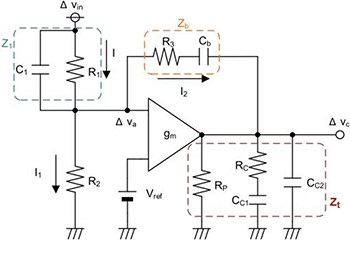Fig. 1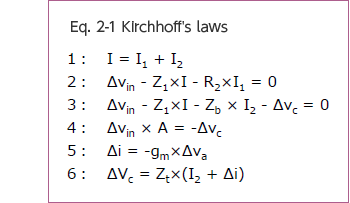Transfer function of an error amplifier

Figure1 is an example of a general error amplifier block. This amplifier compares Vin and Vref, and outputs the results as Vc. Here we derive the transfer function for this amplifier, but first, confirm for yourself that Z1, Zb and Zt are as follows for the circuit blocks surrounded by dashed lines in the diagram.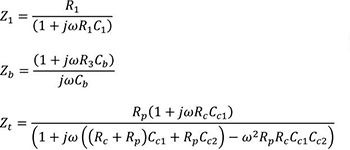Next, Kirchhoff's laws are used to derive the transfer function (Δvc/Δvin) for the amplifier of Fig. 1.

The Kirchhoff laws used in the derivation are shown in eq. 2-1.

Next, the transfer function derived using eq. 2-1 is shown in eq. 2-2.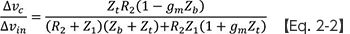Eq. 2-2 can be treated as a general equation for the transfer function of an error amplifier such as that of Fig. 1. Below, eq. 2-2 is used to examine the transfer functions of "a voltage amplifier" and "a current amplifier", which are essential when considering DC/DC converters.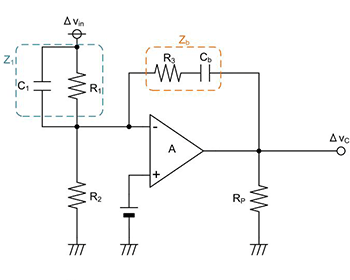Fig. 2

Transfer function of a voltage amplifier

Fig. 2 shows an example of a voltage amplifier configuration. Eq. 2-3 below is a general relationship, appearing on data sheets and the like, for the transfer function of a voltage amplifier.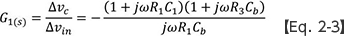We check whether we can derive this eq. 2-3 using the previously derived eq. 2-2. The conditions for eq. 2-2 are as follows.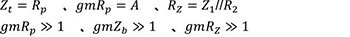Modifying eq. 2-2 based on these conditions, we arrive at eq. 2-4.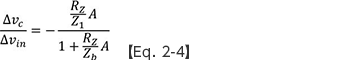Here, when eq. 2-4 satisfies the conditions of eq. 2-5 below, eq. 2-4 can be expressed as eq. 2-6: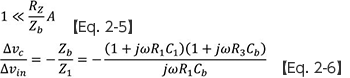Compare this eq. 2-6 with eq. 2-3 above, presented as a general equation for the transfer function of a voltage amplifier. You should be able to see that the two are equivalent.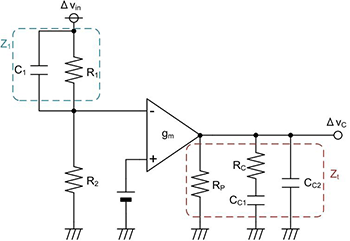Fig. 3

Transfer function of a current amplifier

Next, Fig. 3 shows an example of a current amplifier configuration. Eq. 2-7 below is likewise the equation generally used for the transfer function of this amplifier.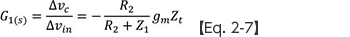Now we similarly attempt to derive eq. 2-7 using eq. 2-2. The conditions for eq. 2-2 are as follows.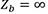Modifying eq. 2-2 based on this, we can derive the following eq. 2-8.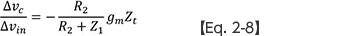Upon comparison with eq. 2-7, it should again be clear that the two are equivalent.

Thus it is seen that transfer functions for a voltage amplifier and a current amplifier can be derived from the transfer function for the error amplifier of Fig. 1 derived in the beginning.